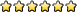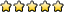## Shock Value B

Jim_R
Admin EmeritusPosts: 283
Joined: May 13th, 2001, 4:22 pm
Division: Grad
Has thanked: 0
Been thanked: 0
Contact:

### Shock Value B

Discussion for Shock Value B.
-The path of the Administrator is beset on all sides by the inequities of the selfish and the tyranny of evil men.

-Nothing\'s gonna get deleted. We\'re gonna be like three little Fonzies here. And what\'s Fonzie like? Come on, what\'s Fonzie like?
-Cool?
-Correctamundo. And that\'s what we\'re gonna be. We\'re gonna be cool. Now, I\'m gonna count to three, and when I count three, you let go of your mouse, and back away from the keyboard. But when you do it, you do it cool. Ready? One... two... three.

gyourkoshaven
MemberPosts: 459
Joined: April 14th, 2008, 12:16 pm
Division: C
State: PA
Has thanked: 0
Been thanked: 0

### Re: Shock Value B

Strath Haven MS:
2008: Regionals-3, States-5
2009: Regionals-2, States-4
2010: Regionals-1, States-2, Nationals-192011 (Co-captain): Regionals-1, States-1, Nationals-11Nationals: Aquifers-37, Compute This-13, Dynamic Planet-25, Ecology-6, Experimental Design-7, Junkyard-33

Moving to C...

blue cobra
Exalted MemberPosts: 417
Joined: April 9th, 2009, 6:10 pm
Division: Grad
State: NY
Has thanked: 0
Been thanked: 0

### Re: Shock Value B

Why does increased resistance mean increased voltage?
In full color since 2006

fleet130
Staff EmeritusPosts: 433
Joined: November 10th, 2001, 3:06 pm
Has thanked: 0
Been thanked: 0
Contact:

### Re: Shock Value B

Ohm's Law states: E = I x R

Where:
R = Resistance in Ohms
E = Electromotive Force in Volts
I = Current in Amperes

If R increases, E must also increase in order for I to remain the same.

In a simple circuit with only 1 EMF source, 1 resistance and 1 current path, increasing the resistance will cause current ( I ) to decrease. In circuits with more than a single resistance in series, each resistance uses (drops) only a portion of the total (applied) voltage. Increasing one of the resistances causes the voltage it drops to increase. Since the applied voltage remains the same, voltage dropped by the other series resistors must decrease.

For Series Circuits:

E(total) = E(R1) + E(R2).... + E(Rn)
R(total) = R1 + R2.... + Rn
I(total) = I(R1) = I(R2).... = I(Rn)

For Parallel Circuits:

E(total) = E(R1) = E(R2).... = E(Rn)
R(total) = 1/[(1/R1) + (1/R2).... + (1/Rn)]
I(total) = I(R1) + I(R2).... + I(Rn)

Edit: Corrected typo in equation for Ohm's Law
Information expressed here is solely the opinion of the author. Any similarity to that of the management or any official instrument is purely coincidental! Doing Science Olympiad since 1987!

Anarch
MemberPosts: 12
Joined: July 2nd, 2009, 1:26 am
Division: C
State: OH
Has thanked: 0
Been thanked: 0

### Re: Shock Value B

You seem to have misstated Ohm's Law. In actuality, Ohm's Law states, using your notation, that E = I x R. Good explanation, otherwise.

To add to that, it is not the case that increasing the resistance in a circuit will increase the voltage. The correct interpretation of this information is that: if you increase the resistance in a circuit, and you want to maintain the same current, you must do something to increase the voltage applied to the circuit (typically by adding a battery or something). A good way to approach this is to treat current as a dependent variable: I = E/R. You cannot do anything to directly change the current flowing through the circuit; rather, you must increase the voltage or decrease the resistance to increase the flow of current (or vice versa for the opposite effect).

andrewwski
Admin EmeritusPosts: 956
Joined: January 12th, 2007, 7:36 pm
Division: Grad
Has thanked: 0
Been thanked: 14 times

### Re: Shock Value B

Correct. If the voltage were constant, increasing the resistance would decrease the current. Increasing the resistance would not increase the voltage on its own under most circumstances, rather it would decrease the current. If one were to adjust the voltage properly, they could maintain the same current load.

fleet130
Staff EmeritusPosts: 433
Joined: November 10th, 2001, 3:06 pm
Has thanked: 0
Been thanked: 0
Contact:

### Re: Shock Value B

You are correct, Ohms Law should be: E = I x R

I have corrected my previous post.
The correct interpretation of this information is that: if you increase the resistance in a circuit, and you want to maintain the same current, you must do something to increase the voltage applied to the circuit
While this is true, I didn't want to increase the applied voltage to maintain the same current. I wanted to show the effect that changing one resistance in a series circuit would have on the circuit.
it is not the case that increasing the resistance in a circuit will increase the voltage.
If only one resistance in a series circuit containing more than one resistance is increased, the voltage drop across THAT resistance WILL increase. Since the applied voltage remains the same, and the sum of the voltages dropped by each resistor is equal to the applied voltage, the voltage dropped by the other resistors in the circuit must decrease. The current will also decrease due to the increase in total resistance.

This change in voltage is easily demonstrated with a few resistors, a battery and a voltmeter.
Information expressed here is solely the opinion of the author. Any similarity to that of the management or any official instrument is purely coincidental! Doing Science Olympiad since 1987!

blue cobra
Exalted MemberPosts: 417
Joined: April 9th, 2009, 6:10 pm
Division: Grad
State: NY
Has thanked: 0
Been thanked: 0

### Re: Shock Value B

I see. Increasing resistance doesn't increase voltage, it decreases current (under most circumstances). Thanks!
In full color since 2006

andrewwski
Admin EmeritusPosts: 956
Joined: January 12th, 2007, 7:36 pm
Division: Grad
Has thanked: 0
Been thanked: 14 times

### Re: Shock Value B

fleet130 wrote:You are correct, Ohms Law should be: E = I x R

I have corrected my previous post.
If only one resistance in a series circuit containing more than one resistance is increased, the voltage drop across THAT resistance WILL increase. Since the applied voltage remains the same, and the sum of the voltages dropped by each resistor is equal to the applied voltage, the voltage dropped by the other resistors in the circuit must decrease. The current will also decrease due to the increase in total resistance.

This change in voltage is easily demonstrated with a few resistors, a battery and a voltmeter.
Correct. When dealing with individual resistors, we can apply Ohms Law to each one, using the information posted above about voltage and current in series and parallel circuits.
blue cobra wrote:I see. Increasing resistance doesn't increase voltage, it decreases current (under most circumstances). Thanks!
Total voltage, no, but voltage through that individual resistor in a series circuit (see fleet's post above). In a parallel circuit, voltage is constant across all branches, so increasing resistance of one or all branches will not affect the voltage through them, just the current. Of course, you're usually going to be dealing with a combination of series and parallel.

Anarch
MemberPosts: 12
Joined: July 2nd, 2009, 1:26 am
Division: C
State: OH
Has thanked: 0
Been thanked: 0

### Re: Shock Value B

fleet130 wrote:
The correct interpretation of this information is that: if you increase the resistance in a circuit, and you want to maintain the same current, you must do something to increase the voltage applied to the circuit
While this is true, I didn't want to increase the applied voltage to maintain the same current. I wanted to show the effect that changing one resistance in a series circuit would have on the circuit.
Oh, I see what you were getting at. That makes sense. Sorry if I seemed overzealous there.

### Who is online

Users browsing this forum: No registered users and 1 guest